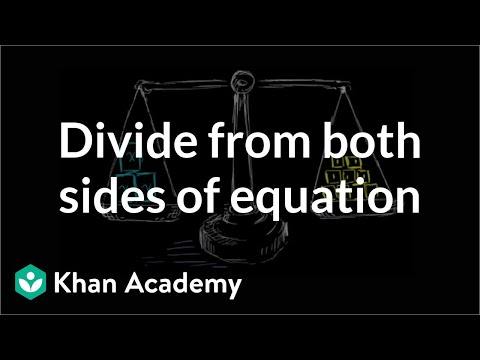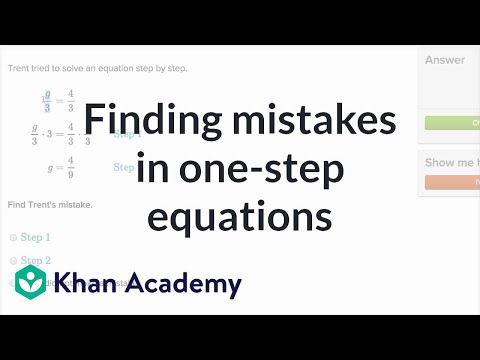Video

# One-step equations intuition (Full video)

Description: This equation can be simplified through a single step to solve for the variable. Can you help? Created by Sal Khan. But what we do know is that if you total up their mass, it's the same exact mass as these nine objects right over here. And each of these nine objects have a mass of 1 kilograms. And then, essentially, we're going to keep things balanced, because we're taking 1/3 of the same mass.

### Other videos you might be interested in### Dividing both sides of an equation (Full video)